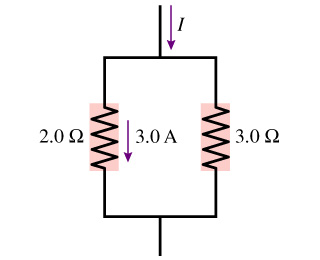# Problem: a. What is the current through the 3.0 Ω resistor?b. What is the value of the current I?

###### FREE Expert Solution

Let's make the following considerations.

79% (69 ratings)###### Problem Detailsa. What is the current through the 3.0 Ω resistor?

b. What is the value of the current I?